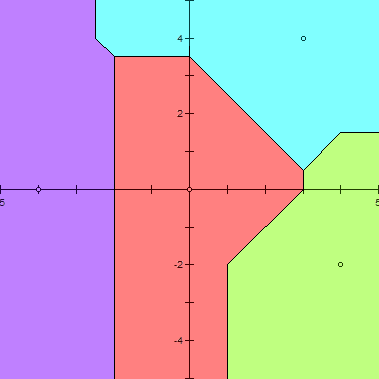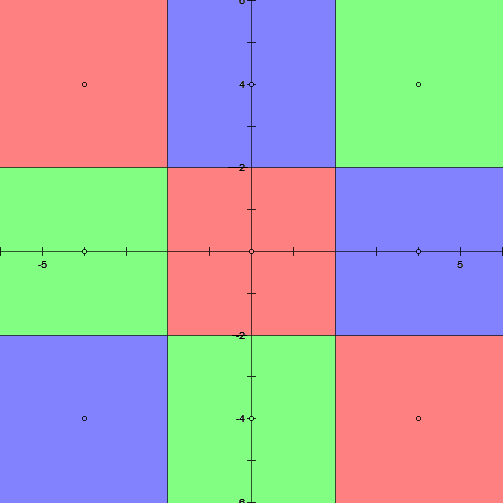시간 제한메모리 제한제출정답맞힌 사람정답 비율
5 초 (추가 시간 없음) 1024 MB182373625.714%

## 문제In the 2-dimensional Cartesian coordinate system, we define the Voronoi Diagram of a non-empty set of points $S$, as a diagram that divides the plane by the criteria which point in the set $S$ is closest in this location?". More precisely, the Voronoi diagram of a given non-empty point set $\{P_1, P_2, \cdots, P_n\}$ is a collection of \textbf{regions}: A point $K$ is included in region $i$ if and only if $d(P_i, K) \le d(P_j, K)$ holds for all $1 \le j \le n$.

While the usual Voronoi Diagram uses Euclidean distance, we use Manhattan distance in this problem. $d(X, Y)$ denotes the \textbf{Manhattan} distance between point $X$ and $Y$. Manhattan distance between two points is the sum of the absolute differences of their $X$, $Y$ coordinates. Thus, the Manhattan distance between two points $(X_1, Y_1)$, $(X_2, Y_2)$ can be written as $|X_2 - X_1| + |Y_2 - Y_1|$.

For example, in the picture above, every location over the plane is colored by the closest point with such location. The points which belongs to a single region is colored by a light color indicating a region, and the points which belongs to more than one region forms lines and points colored black.

The region is unbounded if for any real number $R$, there exists point $P$ in the region such that $d(O, P) > R$ where $O$ is the origin. You have to find the number of unbounded regions in the Voronoi Diagram.

## 입력

In the first line, the number of points consisting Voronoi diagram $N$ is given.

In the $i$-th line of next $N$ lines, two integers $x_i,\ y_i$ indicating $x$ and $y$ coordinate of $P_i$ are given. These are the points in the Voronoi diagram.

## 출력

Print a single integer, denoting the number of unbounded regions in the Voronoi Diagram.

## 제한

• $1 \le N \le 250\,000$
• $-10^9 \le x_i,\ y_i \le 10^9$ ($1 \le i \le N$)
• All $N$ points are distinct.

## 서브태스크 1 (15점)

• $N \le 2\,000$
• $-100 \le x_i,\ y_i \le 100$ ($1 \le i \le N$)

## 서브태스크 2 (35점)

• $N \le 2\,000$

## 예제 입력 1

4
0 0
-4 0
3 4
4 -2

## 예제 출력 1

4## 예제 입력 2

5
1 1
2 2
3 3
4 4
5 5

## 예제 출력 2

5## 예제 입력 3

9
-4 -4
-4 0
-4 4
0 -4
0 0
0 4
4 -4
4 0
4 4

## 예제 출력 3

8## 힌트

In example 2, overlapping region is indicated as subtractive mixing of two or more colors. All points with $(x \ge 5 \wedge y \le 1) \vee (x \le 1 \wedge y \ge 5)$ is included in all five region, and colored as darkest.

## 채점 및 기타 정보

• 예제는 채점하지 않는다.